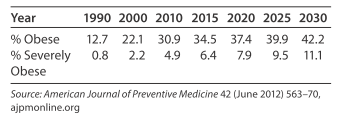# Modeling Obesity Obesity ( BMI ≥ 30 ) is a serious problem in the United States and expected to get worse. Being overweight increases the risk of diabetes, heart disease, and many other ailments, but the severely obese ( BMI ≥ 40 ) are most at risk and are the most expensive to treat. The percent of Americans who are obese and severely obese from 1990 projected to 2030 are shown in the table below. Report the following models with three significant digits. Find the linear function, O ( x ) , that models the percent of Americans who are obese, with x equal to the number of years past 1980. Find the linear function, S ( x ) , that models the percent of Americans who are severely obese, with x equal to the number of years past 1980. Form the rational function F ( x ) that gives the fraction of obese Americans who are severely obese. Find lim x → ∞ F ( x ) and tell what it means in terms of these data. What does part (d) tell us about the graph of y = F ( x ) ? Does F ( x ) have any vertical asymptotes for x &gt; 0 ?### Mathematical Applications for the ...

11th Edition
Ronald J. Harshbarger + 1 other
Publisher: Cengage Learning
ISBN: 9781305108042

#### Solutions

Chapter
Section### Mathematical Applications for the ...

11th Edition
Ronald J. Harshbarger + 1 other
Publisher: Cengage Learning
ISBN: 9781305108042
Chapter 10.5, Problem 44E
Textbook Problem
1 views

## Modeling Obesity Obesity ( BMI ≥ 30 ) is a serious problem in the United States and expected to get worse. Being overweight increases the risk of diabetes, heart disease, and many other ailments, but the severely obese ( BMI ≥ 40 ) are most at risk and are the most expensive to treat. The percent of Americans who are obese and severely obese from 1990 projected to 2030 are shown in the table below. Report the following models with three significant digits. Find the linear function, O ( x ) , that models the percent of Americans who are obese, with x equal to the number of years past 1980. Find the linear function, S ( x ) , that models the percent of Americans who are severely obese, with x equal to the number of years past 1980. Form the rational function F ( x ) that gives the fraction of obese Americans who are severely obese. Find lim x → ∞ F ( x ) and tell what it means in terms of these data. What does part (d) tell us about the graph of y = F ( x ) ? Does F ( x ) have any vertical asymptotes for x > 0 ?This textbook solution is under construction.

### Still sussing out bartleby?

Check out a sample textbook solution.

See a sample solution

#### The Solution to Your Study Problems

Bartleby provides explanations to thousands of textbook problems written by our experts, many with advanced degrees!

Get Started